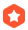# 指针的基本操作和实际应用星落化尘 2023-11-18 19:07:26加精

## 2. 基本使用

### 2.1 普通指针

``````//输出语句用的是C++的输出，理解不了全都替换成下面的式子

printf("%d",p);

//----------
//定义一个int类型变量
//----------

int a = 0;
//这个时候我们输出这个a获得的结果式0

//----------
//定义一个int类型的指针变量，并赋值
//----------

//错误写法：
int *p = 0;
//这样的定义含义是：一个指针他指向了地址为0的区域

//正确写法：
int *p = &a;
//指向a地址的指针

//----------
//定义一个指针变量，并初始化
//----------

int *p = (int*)malloc(sizeof(int));
//这样定义的含义是：系统分配一个空间给指针p，但他的初始值是未知的

//----------
//指针的输出
//----------

cout << p;
//等价于
cout << &a;
//输出a的地址

cout << *p;
//等价于
cout << a;
//输出a的值

``````

1. 前两个输出是否相等

2. 后两个输出是否相等

``````#include<iostream>
using namespace std;

int main() {
int a = 1;

int* p= (int*)malloc(sizeof(int));
*p = a;

cout << p << endl;
cout << &a << endl;

p = &a;
cout << p << endl;
cout << &a << endl;
}``````

总结（八个字）：p是地址，*p是数据

``````//首先定义一个数组

int a[] = {1, 2, 3, 4};

//如果我们直接输出
cout << a;    //1

//这个结果大家都知道，但是
cout << a;

//他的结果是a数组的首位地址：000000CB7031F678
//每次运行结果不一样的

//所以我们现在有个猜测, 没有这个想法的翻到上面基础测试再看看

int *p = a;

cout << p;    //依旧是输出首地址

cout << *p;
cout << *a;   //结果会是一样的，都是  1

cout << p;    //也是可以的

cout << ++p;    //是可以实现的
cout << ++a;    //这个写法是不被允许的！！！！！！！！！！！！！这个为什么不行等下再说

//我们嫩继续开发p的用法
p++；
//在这步运算之后
cout << *p;    //这个值就不是1了，而是：2

//这个时候
cout << p[-1];    //这个输出结果是：1

//应用

//遍历数组
int *p = a;
for(int i=0;i<4;i++){
cout << *p << endl;
//endl 相当于 \n
p++;
}
``````

``````int a = {1,2,3,4};

//数组地址：其实就是第一个元素的位置，所以

cout << a;
cout << &a;
cout << &a;

//上述是一样的

//获取第二个元素的地址
cout << &&a;``````

## 2.2 数组指针

``````//一样还是定义一个数组
int a = {1,2,3,4};

//定义数组指针

int (*p) = &a;    //这里这个a是和前面的不同的哦，需要使用取址符

//输出
cout << p << endl;    //他获取的是这个数组的首址
cout << *p << endl;    //和上面那个一样
cout << p << endl;    //他获取的是指针指向的地址

//这几个输出的结果都是地址，原因我写在上面

//这时判断一下这个

cout << p << endl;
//大家觉得是 p+4 还是 p+16 呢
//实际情况是 +16

//这个时候，帅气的学弟和漂亮的学妹们应该会问：“我的数据跑哪去了？”

//在这里
cout << **p << endl;    //1

//他变成一个二维数组啦

cout << p << endl;    //2

``````

### 2.3 指针数组

``````//其实就是一个很普通的二维数组
int a = { 1,2,3,4 };
int b = { 5,6,7,8 };
int* p;
p = a;
p = b;
int c = 0;
int d = 1;
p = &c;
p = &d;
for (int i = 0; i < 4; i++) {
for (int j = 0; j < 4; j++) {
cout << p[i][j] << " ";
}
cout << endl;
}

//这是输出结果，其实本质和前面的是一样的
//所以我就不分析这个了，看不懂的话
//前面的两种分析多看看
//1 2 3 4
//5 6 7 8
//0 -858993460 -858993460 -858993460
//1 -858993460 -858993460 -858993460

//这几个负数是因为这里没有定义数据

//需要注意的是，p是个空指针，如果输出了就爆栈，段错误``````

### 2.4 字符指针

``````//上一期的博客是不是有一个错误的写法是

char a = "1234";

//但是可以这么写

char* a = "1234";

//虽然这个是可以的，但是过不了vs的编译器

//输出数据
printf("%c",*a);    //1
printf("%s",a);    //1234

//特殊的输出
cout << *(a+2);    //3    %c
cout << a + 2;    //34    %s

//输出地址
cout << &a;    //这个没什么特别的，所以还是C++写

//修改字符

a = 5;
cout << a;    //大家可能会认为是：1534
//但是实际上这个写法是错误的--段错误
//其实是非法访问了地址，因为这个字符指针是常量无法修改

//最好的定义方法
const char* a = "1234";

//虽然不难修改部分字符，但是可以修改整个串

a = "1534";    //字符串变了，但是地址不变

//这里确实是覆盖了
//内部原理比较复杂，这里大家自己去查常量区的相关知识

``````

1. 字符串数组是可以对字符进行操作的, 正确演示： a = 5;

2. 字符串数组不可以直接将字符串赋值给字符串数组, 错误演示：a = "1534";

### 2.5 指针函数

``````#include<stdio.h>

void change(int a,int b){
int temp = a;
a = b;
b = temp;
}

int main(){
int a = 1;
int b = 2;
printf("%d %d\n",a,b);
change(a, b);    //英语就这水平，问题不大
printf("%d %d",a,b);
}

//输出结果：
//1 2
//1 2``````

``````#include<stdio.h>

void change(int* a,int* b){    //这里的*要加
int temp = *a;
*a = *b;
*b = temp;
}

int main(){
int a = 1;
int b = 2;
printf("%d %d\n",a,b);
change(&a, &b);    //这里的&要加
printf("%d %d",a,b);
}

//输出结果：
//1 2
//2 1

//传入的数据是地址，所以其内部都是对该内部的数据进行交换
//同样也是后续会写博客介绍``````

``````#include<stdio.h>

int a,b;

void change(){
int temp = a;
a = b;
b = temp;
}

int main(){
a = 1;
b = 2;
printf("%d %d\n",a,b);
change();    //英语就这水平，问题不大
printf("%d %d",a,b);
}

//输出结果
//1 2
//2 1

//注意啊，函数是没有输入的，如果你把a, b输入其中，那么函数只会交换在里面的数据，和全局变量不同
//这些细节的东西应该也是之后会出一篇对变量的介绍``````

### 2.6 后续学习方向

STL（C++的很重要的容器库，如果想要学习可以看黑马程序员C++课程的后半部，大家也可以问问自己组的助教学长！）

...全文
9 回复 打赏 收藏 转发到动态 举报C++ 11 14 17 20内存管理-指针、智能指针和内存池单链表的基本操作应用c语言指针用法及实际应用详解，通俗易懂超详细！指针在函数中的应用指针和引用的常规用法以及应用总结

180发帖与我相关我的任务
c语言c++ 高校 福建省·厦门市• 近7日
• 近30日
• 至今